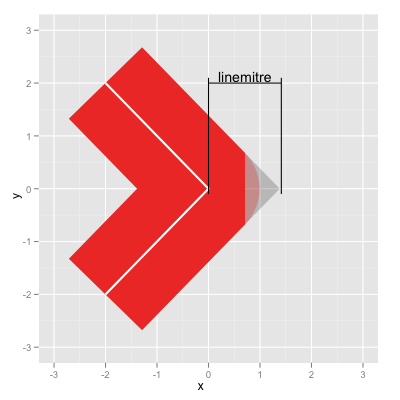## ggplot2 Quick Reference: linemitre

Note that `linemitre` is a parameter to certain (but not all) geoms that draw lines, but it is not an aesthetic (that is, it can not be used in a mapping via a scale but can only be set to a constant value for any given layer).

### Setting to constant value

The `linemitre` parameter only makes sense for lines with a "mitre" linejoin. It accepts a numerical value greater than or equal to 1. It represents the limit by which a mitre join (`linejoin="mitre"`) can extend from the line's point. If the mitre join extends beyond that limit, then it is turned into a butted join (`linejoin="butt"`). The limit is specified as a fraction of half the line-width. Thus, a limit of 1 is equivalent to the extent of a rounded join (`linejoin="round"`).```d=data.frame(x=c(-2,0,-2), y=c(-2,0,2))
ggplot() +
geom_path(data=d, mapping=aes(x=x, y=y), size=48, alpha=0.2, linejoin="mitre", linemitre=sqrt(2)) +
geom_path(data=d, mapping=aes(x=x, y=y), size=48, linejoin="round", color="red", alpha=0.3) +
geom_path(data=d, mapping=aes(x=x, y=y), size=48, linejoin="bevel", color="red") +
geom_path(data=d, mapping=aes(x=x, y=y), size=1, color="white") +
scale_x_continuous(limits=c(-3,3)) +
scale_y_continuous(limits=c(-3,3)) +
geom_segment(mapping=aes(x=0, y=2, xend=sqrt(2), yend=2)) +
geom_segment(mapping=aes(x=sqrt(2), y=2.1, xend=sqrt(2), yend=-0.1)) +
geom_segment(mapping=aes(x=0, y=2.1, xend=0, yend=-0.1)) +
geom_text(mapping=aes(x=sqrt(2)/2, y=2, label="linemitre"), vjust=-0.2)```

Note that this page just reflects our guess of the meaning of this parameter. It is based on trial and error.